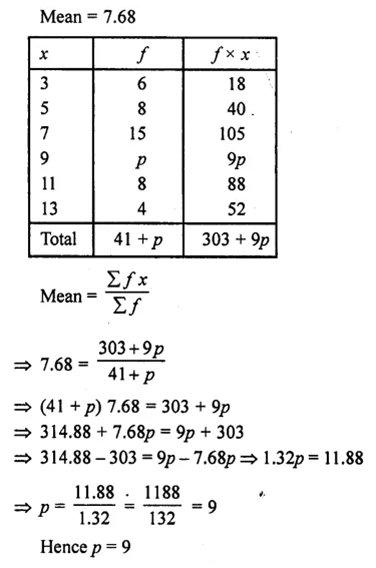• +91 9971497814
• info@interviewmaterial.com

# RD Chapter 7- Statistics Ex-7.1 Interview Questions Answers

### Related Subjects

Question 1 : Calculate the mean for the following distribution :
 X 5 6 7 8 9 f 4 8 14 11 3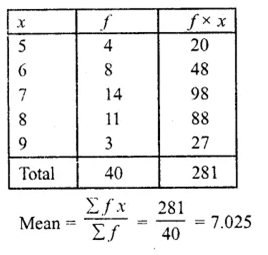Question 2 : Find the mean of the following data:
 X 19 21 23 25 27 29 31 f 13 15 16 18 16 15 13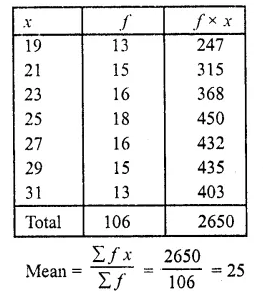Question 3 :

If the mean of the following data is 20.6. Find the value of p. (C.B.S.E.1997)

 X 10 15 p 25 35 y 3 10 25 7 5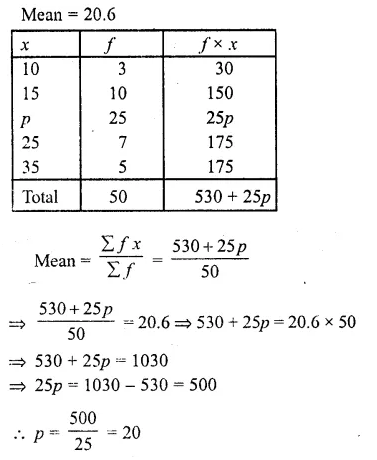Question 4 : If the mean of the following data is 15, find p. (C.B.S.E. 1992C)
 X 5 10 15 20 25 f 6 P 6 10 5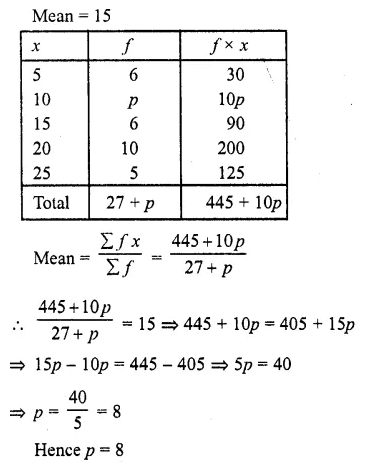Question 5 : Find the value of p for the following distribution whose mean is 16.6.
 X 8 12 15 P 20 25 30 f 12 16 20 24 16 8 4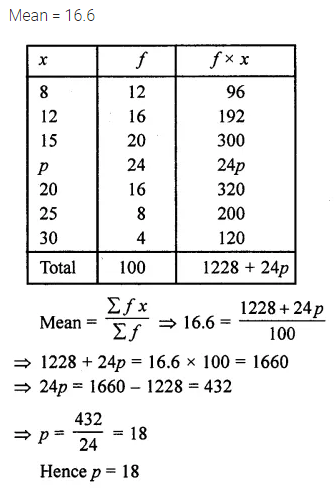Question 6 : Find the missing value of p for the following distribution whose mean is 12.58. (C.B.S.E. 1992C)
 X 5 8 10 12 P 20 25 f 2 5 8 22 7 4 2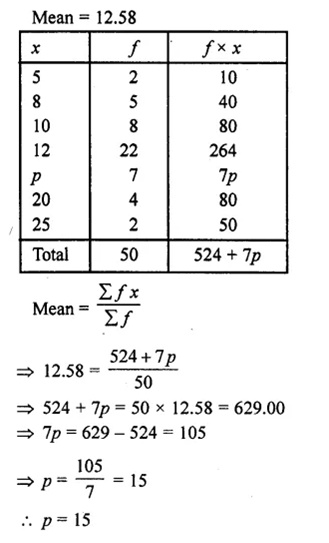Question 7 : Find the missing frequency (p) for the following distribution whose mean is 7.68.
 X 3 5 7 9 11 13 f 6 8 15 P 8 4